# Use the following pair of reduction half-reactions to design a galvanic cell. Then write in the...

Use the following pair of reduction half-reactions to design a galvanic cell. Then write in the proper coefficient for each of the species involved in the overall reaction. Water molecules and protons are not shown in the half-reactions, but may be needed in the overall reaction.

 MnO4- (aq) → MnO2 (s) ξo = 0.59 V NO3- (aq) → NO (g) ξo = 0.96 V

NO3- (aq)
MnO4- (aq)
NO (g)
MnO2 (s)
H+ (aq)
H2O (l)#### Earn Coin

Coins can be redeemed for fabulous gifts.

Similar Homework Help Questions
• ### Use the following pair of half-reactions to design a galvanic cell. Then wite in the proper...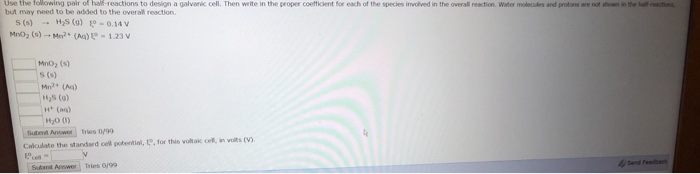Use the following pair of half-reactions to design a galvanic cell. Then wite in the proper coeffickent for each of the species involved in the overall reaction. Water molecules and protons are not shoan in the hall but may fneed to be added to the overall reaction s (s) H2S (g) e-0.149 → MnO2 (s) s (s) Mn (A “JS (0) H (on) H20 (0) Caiculate the standard cell potertial, 1,for this vollak cefl, in volits (V) Tries ojgo

• ### A chemist designs a galvanic cell that uses these two half-reactions: half-reaction standard reduction potential Ed=+0.96...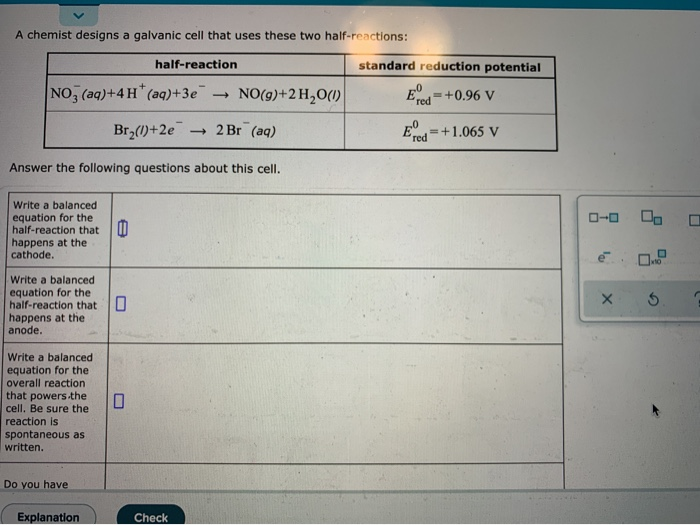A chemist designs a galvanic cell that uses these two half-reactions: half-reaction standard reduction potential Ed=+0.96 v NO3(aq)+4 H* (aq)+3e NO(g)+2H2O() Br2()+2e → 2 Br (aq) Erd- +1.065 V Answer the following questions about this cell. 0-0 0 0 Write a balanced equation for the half-reaction that happens at the cathode. Write a balanced equation for the half-reaction that happens at the anode. Write a balanced equation for the overall reaction that powers the cell. Be sure the reaction is...

• ### A chemist designs a galvanic cell that uses these two half-reactions: half-reaction standard reduction potential Zn(s)...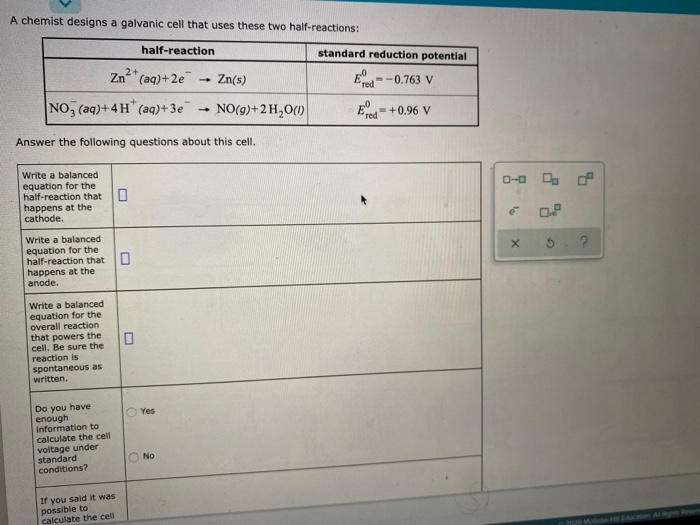A chemist designs a galvanic cell that uses these two half-reactions: half-reaction standard reduction potential Zn(s) red -0.763 V Zn2+ (aq)+2e NO3(aq)+4 H+ (aq)+3e NO(9)+2H20(1) EO red = +0.96 V Answer the following questions about this cell. Write a balanced equation for the half-reaction that happens at the cathode. е х Write a balanced equation for the half-reaction that happens at the anode. Write a balanced equation for the overall reaction that powers the cell. Be sure the reaction is...

• ### A chemist designs a galvanic cell that uses these two half-reactions: half-reaction standard reduction potential Ed=-0.763...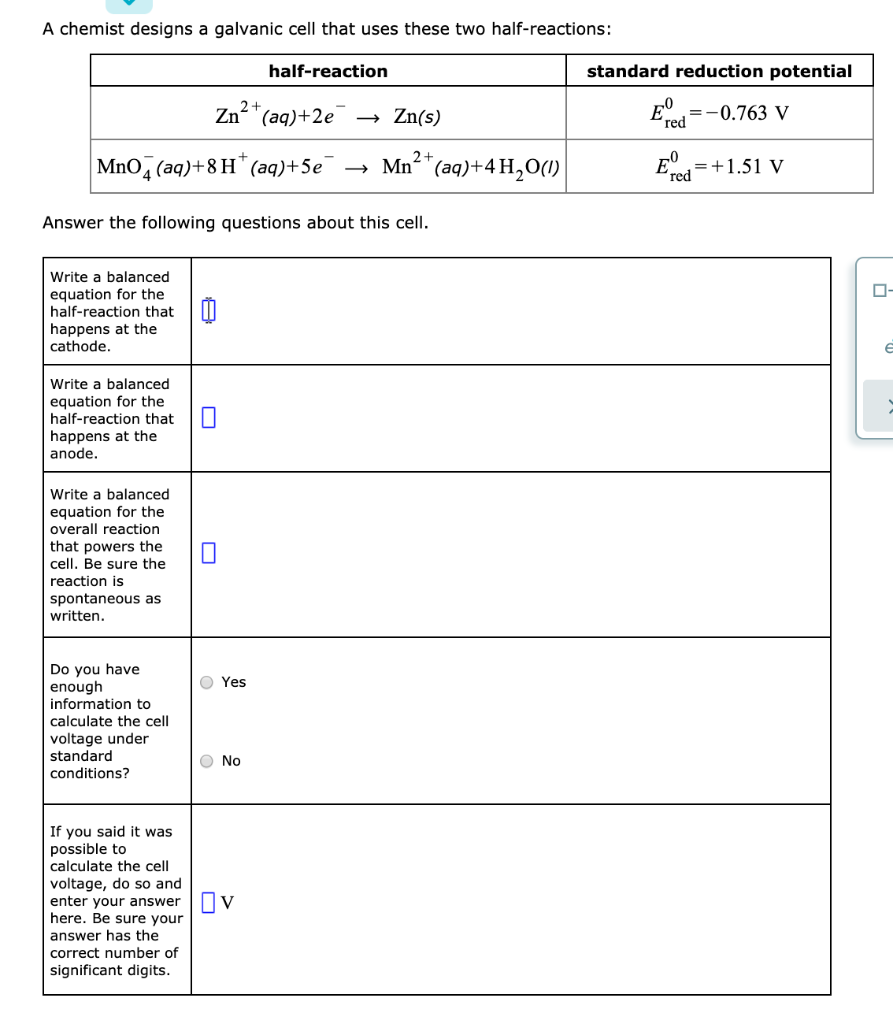A chemist designs a galvanic cell that uses these two half-reactions: half-reaction standard reduction potential Ed=-0.763 V Zn²+(aq)+2e MnO2 (aq)+8H+ (aq)+5€ → Zn(s) → Mn²+ (aq)+4 ,00) E cd = +1.51 v Answer the following questions about this cell. Write a balanced equation for the half-reaction that happens at the cathode. Write a balanced equation for the half-reaction that happens at the anode. Write a balanced equation for the overall reaction that powers the cell. Be sure the reaction is...

• ### A chemist designs a galvanic cell that uses these two half-reactions half-reaction standard reduction potential red...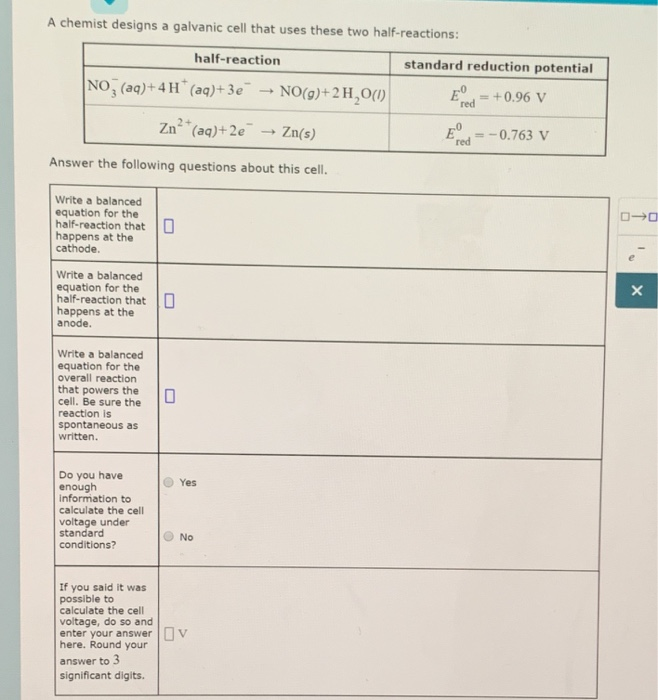A chemist designs a galvanic cell that uses these two half-reactions half-reaction standard reduction potential red = +0.96 V red0.763 v NO, (ag)+4H(a)+3eNoo)+2 H,Ow Zan2+ (aq)+2e- → Zn(s) Answer the following questions about this cell Write a balanced equation for the half-reaction that happens at the cathode Write a balanced equation for the half-reaction that happens at the anode Write a balanced equation for the overall reaction that powers the cell. Be sure the reaction is spontaneous as written. Do...

• ### A chemist designs a galvanic cell that uses these two half-reactions: standard reduction potential half-reaction +...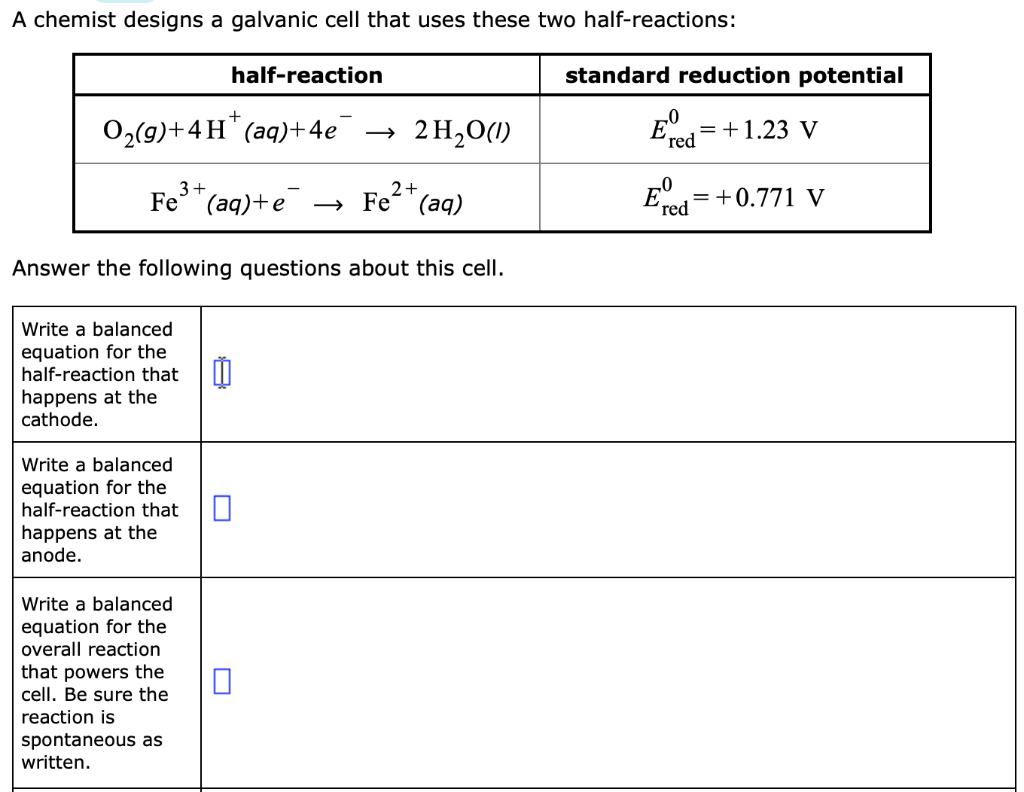A chemist designs a galvanic cell that uses these two half-reactions: standard reduction potential half-reaction + O2(9)+4 H (aq)+4e' 2H20) = 1.23 V red Ered Fe+. (аq) Fe3(aq)+e = +0.771 V Answer the following questions about thiss cell Write a balanced equation for the half-reaction that happens at the cathode Write a balanced equation for the half-reaction that happens at the anode Write a balanced equation for the overall reaction that powers the cell. Be sure the reaction is spontaneous...

• ### A chemist designs a galvanic cell that uses these two half-reactions: half-reaction standard reduction potential Ecd=-0.13...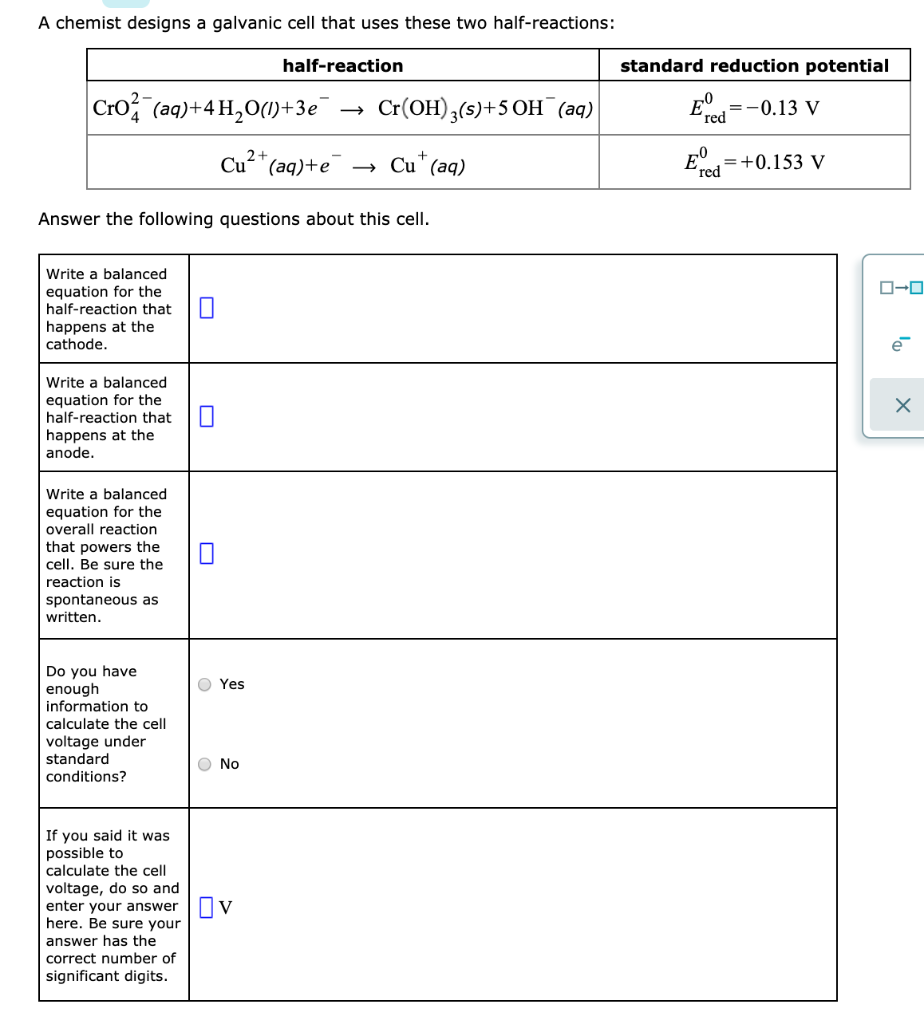A chemist designs a galvanic cell that uses these two half-reactions: half-reaction standard reduction potential Ecd=-0.13 V Cro2 (aq)+4 H2O(1)+3e" → Cr(OH)3(s)+50H (aq) Cu2+ (aq)+e → Cut(aq) = +0.153 V Answer the following questions about this cell. 0-0 Write a balanced equation for the half-reaction that happens at the cathode. DI X Write a balanced equation for the half-reaction that happens at the anode. Write a balanced equation for the overall reaction that powers the cell. Be sure the reaction...

• ### Balance the following oxidation-reduction reactions using the half-reaction method. 1. HCOOH (aq) + MnO.. (aq) → CO2 (g) + Mn2. Acidie solution Identify the reduction half Identify the oxidation...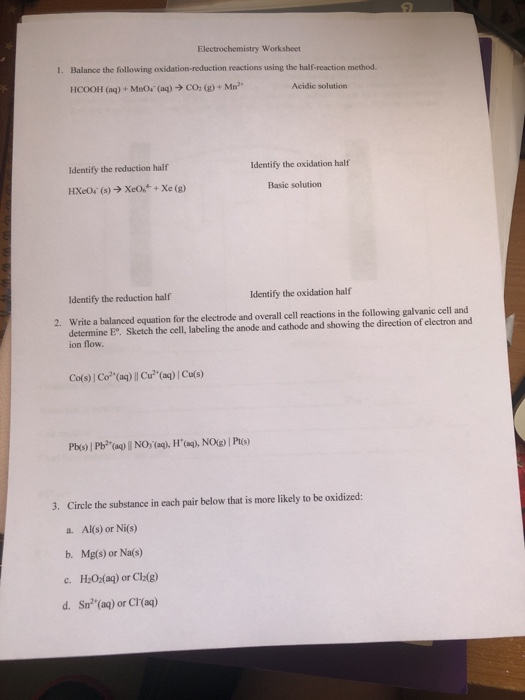Balance the following oxidation-reduction reactions using the half-reaction method. 1. HCOOH (aq) + MnO.. (aq) → CO2 (g) + Mn2. Acidie solution Identify the reduction half Identify the oxidation half Basic solution Identify the reduction half Identify the oxidation half Write a balanced equation for the electrode and overall cell reactions in the following galvanic cell and determine E°. Sketch the cell, labeling the anode and cathode and showing the direction of electron and ion flow. 2. 3. Circle the...

• ### A chemist designs a galvanic cell that uses these two half-reactions: half-reaction standard reduction potential Eped...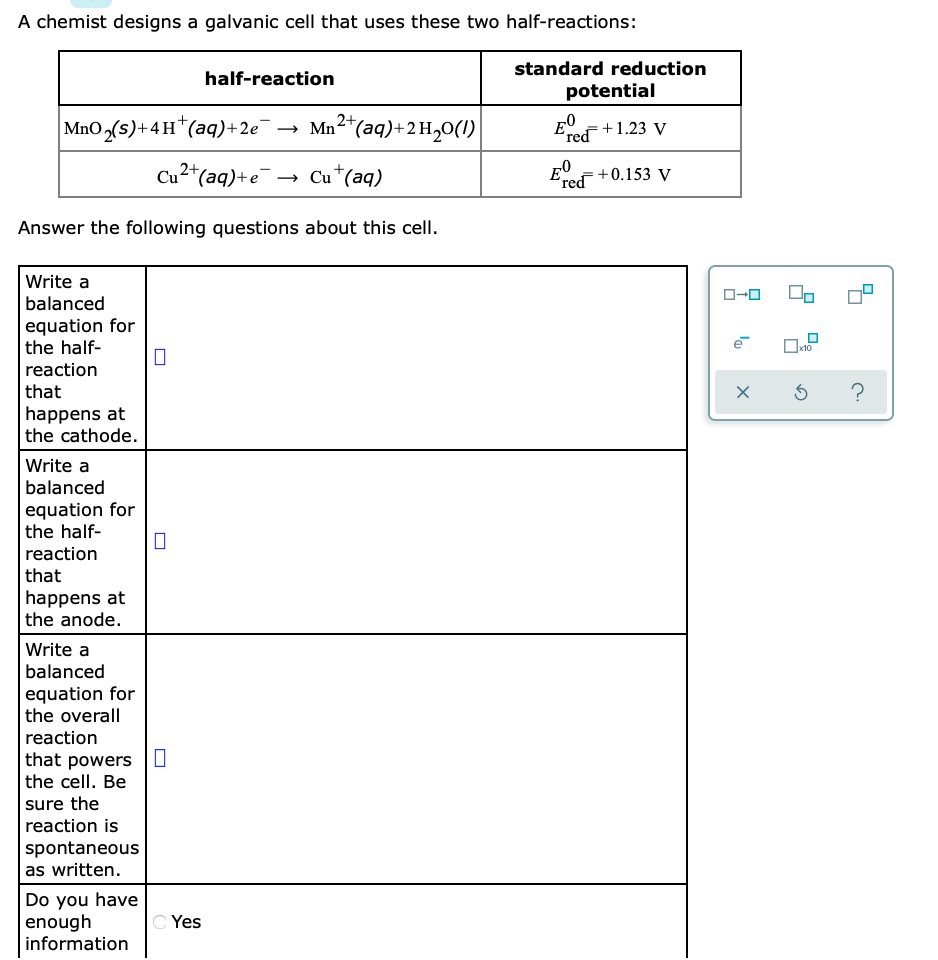A chemist designs a galvanic cell that uses these two half-reactions: half-reaction standard reduction potential Eped +1.23 V MnO,(s)+44*(aq)+2e → Mn2+(aq)+2H20(1) Cu2+(aq)+e → Cut(aq) Eped +0.153 V Answer the following questions about this cell. xs ? Write a balanced equation for the half- reaction that happens at the cathode. Write a balanced equation for the half- reaction that happens at the anode. Write a balanced equation for the overall reaction that powers the cell. Be sure the reaction is spontaneous...

• ### A chem igns a galvanic cell that uses these two half reactions: standard reduction potential half-reaction...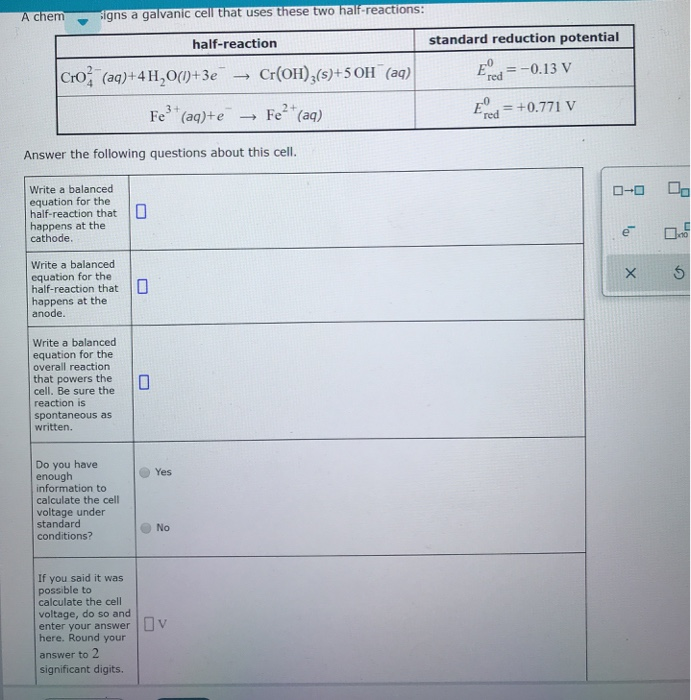A chem igns a galvanic cell that uses these two half reactions: standard reduction potential half-reaction (aq)+4 H,0(1)+3e" → Cr(OH)2(3)+50H (aq) cro Ered=-0.13 V Fe?+ (aq)+e → Fe2+ (aq) E = +0.771 V Answer the following questions about this cell. 0-0 0 Write a balanced equation for the half-reaction that happens at the cathode. Write a balanced equation for the half-reaction that happens at the anode. X 5 Write a balanced equation for the overall reaction that powers the cell....